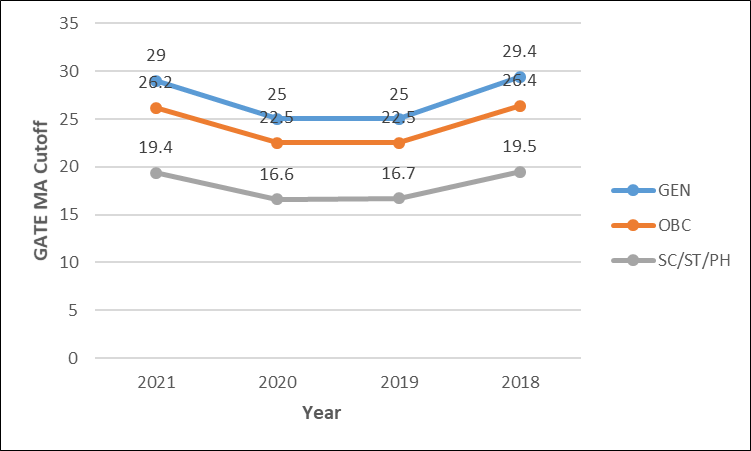# GATE Mathematics

IIT Kanpur will conduct the GATE Mathematics (MA) 2023 Exam. GATE 2023 Mathematics Paper had a total of 65 questions. Of these total questions, 55 questions were on Mathematics, and 10 questions were on the General Aptitude section. The candidates who opt for Mathematics as their first paper in GATE Exam can also appear for Computer Science and Information Technology (CS), Geomatics Engineering (GE), Physics (PH), or Statistics (ST) as their second choice of paper in GATE 2023.

Get all the necessary information like syllabus, exam pattern, new changes introduced in GATE, important books, preparation tips, previous years’ question papers, cut off, and latest news related to GATE Mathematics Exam here. We have also provided direct links to the Answer Key and Question Papers.

## GATE Maths Latest News

GATE 2023 Mathematics aspirants can now access the GATE Syllabus and GATE Exam Dates on the official website.

### GATE Maths Overview

 Particulars Details Number of Questions in Paper 65 Number of Sections Section 1 – General Aptitude, Section 2 – Mathematics and Computer Science Engineering Topic-wise Weightage General Aptitude – 15% Mathematics – 85% Pattern of Questions Multiple Choice Question (MCQs) and Numerical Answer Type Questions (NATQs) Duration of Exam 3 Hours Marking Scheme Test paper has questions carrying 1 mark and 2 marks, and ⅓ and ⅔ negative mark for incorrect answers, respectively

## GATE Mathematics Related Study Resources

### Books for GATE Maths

Some of the important books for GATE MA are listed below:

 Subject Book Title Author Linear Algebra Linear Algebra Seymour Lipschutz, Marc Lipson Linear Algebra and its Applications Gilbert Strang Complex Analysis Complex Analysis Gamelin Complex Analysis for Mathematics and Engineering J. H. Mathews Foundations of Complex Analysis S. Ponnusamy Real Analysis Real Analysis Royden H.L., Fitzpatrick P. M Introduction to Real analysis Donald R. Sherbert, Robert G. Bartle Elements of Real Analysis Shanti Narayan, M D Raisinghania Ordinary Differential Equations Ordinary Differential Equations Purna Chandra Biswal An Introduction to Ordinary Differential Equations Earl A. Coddington Ordinary and Partial Differential Equations M. D. Raisinghania Partial Differential Equations Ordinary and Partial Differential Equations M. D. Raisinghania Elements of Partial Differential Equations Ian N. Sneddon Introduction to Partial Differential Equations Sankara Rao Algebra Topics in Algebra I. N. Herstein Linear Algebra Ian N. Sneddon, Seymour Lipschutz, Marc Lipson Functional Analysis Functional Analysis Rudin Introductory Functional Analysis with Applications Erwin Kreyszig Numerical Analysis Numerical Analysis Francis Scheid Introductory Methods of Numerical Analysis Sastry S. S. Numerical Methods – Principles, Analysis & Algorithms Srimanta Pal Mechanics Classical Mech John Safko, Charles P. Poole Herbert Goldstein Engineering Mechanics S. S. Bhavikatti Topology Topology James R. Munkres Introduction to Topology and Modern Analysis S. S. Bhavikatti Probability and Statistics Probability and Statistics John J. Schiller Introduction to Probability and Statistics for Engineers and Scientists Sheldon M. Ross Probability, Random Variables and Stochastic Processes S Pillai, Athanasios Papoulis Linear Programming Linear Programming G. Hadley, J.G Chakraborty & P. R. Ghosh Linear Programming and Network Flows Mokhtar S. Bazaraa, John J. Jarvis, Hanif D. Sherali Calculus of Variation and Integral Equations An Introduction to the Calculus of Variations Pars Calculus of Variations I. M. Gelfand, Wendy Ed. Silverman Integral Transforms, Integral Equations, and Calculus of Variations P. C. Bhakta

## GATE Maths Important Dates 2023

 Particulars Dates GATE online application processing 30th August, 2022 Closing of submission of application form 30th September, 2022 Last date of the extended period for online registration application (with late fees) 7th October, 2022 Modifications in GATE 2023 Application 4th to 11th November, 2022 Admit Card 3rd January, 2023 GATE 2023 examination 4th, 5th, 11th and 12th February, 2023 Announcement of result 16th March, 2023

## GATE Maths (MA) Exam Pattern

Let us take a look at the GATE Exam pattern for the 2022 exam for Maths:

 Feature Description Examination Mode Online (Computer Based Test) Duration of Exam 3 Hours (180 Minutes) Sectional Time Duration None Total Marks 100 Total Number of questions 65 Number of Subjects (Papers) 29 Number of Sections It depends on the subjects. 2-3 (GA and Core Discipline or GA, Engineering Mathematics and Core Subject) Type of Questions Multiple Choice Questions (MCQs) Multiple Select Questions (MSQs) Numerical Answer Type (NAT) Questions Marking Scheme 1 or 2 marks for each right answer Negative Marking Scheme For a wrong answer chosen in a MCQ, there will be negative marking. For 1-mark MCQ, 1/3 mark will be deducted for a wrong answer. For 2-mark MCQ, 2/3 mark will be deducted for a wrong answer. NO negative marking for MSQ & NAT.

Marking Scheme of GATE MA:

 Stream/ Course Subject Total No. of Questions Marks Allotted Mathematics General Aptitude 10 15 Subjects 55 85 Total 65 100

## GATE Mathematics Paper Analysis

IIT Kanpur will conduct the GATE Maths 2023 exam on February, 2023. You can find more details below:

 Particulars Details Question Type MSQs NATQs MCQs Difficulty Level Moderate Highest weightage in the paper Topology Calculus Ode and Pde Real Analysis General Aptitude Moderate

## GATE Mathematics Results Analysis

### GATE MA Topper Marks by Year

 Year Subject Marks 2021 Maths (MA) 68.33 2020 Maths (MA) 61.0 2019 Maths (MA) 48.33 2018 Maths (MA) 49.67 2017 Maths (MA) 57.67

### GATE MA Cutoff over Years

 Year Subject GEN OBC SC/ST/PH 2021 Maths (MA) 29 26.2 19.4 2020 Maths (MA) 25 22.5 16.6 2019 Maths (MA) 25 22.5 16.7 2018 Maths (MA) 29.4 26.4 19.5

The graph shows the MA cutoff trend of the last 5 years:Q1

### Who is eligible for GATE mathematics?

Anyone with a Master’s degree in any branch of Science/Mathematics/Statistics/Computer Applications or equivalent are eligible for GATE Mathematics.

Q2

### How many attempts are there for GATE?

There is no limit for appearing GATE as per GATE 2023 notifications.

Q3

### What is a good rank in GATE mathematics?

To get admission in top IITs, a rank below 200 is necessary.